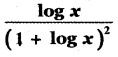Tamilnadu State Board New Syllabus Samacheer Kalvi 11th Maths Guide Pdf Chapter 11 Integral Calculus Ex 11.9 Text Book Back Questions and Answers, Notes.

## Tamilnadu Samacheer Kalvi 11th Maths Solutions Chapter 11 Integral Calculus Ex 11.9

Integrate the following with respect to x:

Question 1.
ex (tan x + log sec x)
Let I = ∫ex (tan x + log sec x) dx
Take f(x) = log sec x
f'(x) = $$\frac{1}{\sec x}$$ sec x tan x
f'(x) = tan x
[∫ex [f (x) + f (x)] dx = ex f(x) + c]
∴ I = ex log |sec x| + cQuestion 2.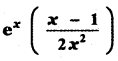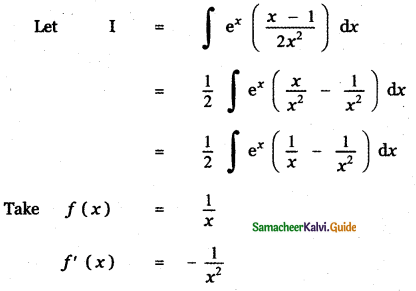[∫ ex [f (x) + f (x)] dx = ex f(x) + c]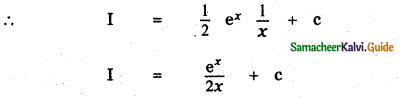Question 3.
ex sec x (1 + tan x)
Let I = $$\mathrm{I}=\int e^{x}(\sec x+\sec x \tan x) d x$$
Take f(x) = sec x
f ‘ (x) = sec x tan x
This is of the form of $$\int e^{x}\left[f(x)+f^{\prime}(x)\right] d x$$ = ex f(x) + c
= ex sec x + c

Question 4.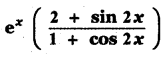Take f(x) = tan x
f'(x) = sec2 x
[∫ ex [f (x) + f (x)] dx = ex f(x) + c]
I = ex tan x + cQuestion 5.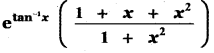Put tan-1 x = t
$$\frac{1}{1+x^{2}}$$dx = dt
x = tan t
∴ I = ∫et [1 + tan t + tan2t] dt
= ∫et [1 + tan2 t + tan t] dt
= ∫et [sec2t + tan t] dt
= ∫et [tan t + sec2t] dt
f(x) = tan t
f'(x) = sec2t
[∫ ex [f (x) + f (x)] dx = ex f(x) + c]
∴ I = et tan t + c
I = etan-1(x) . x + c
I = x etan-1(x) + cQuestion 6.